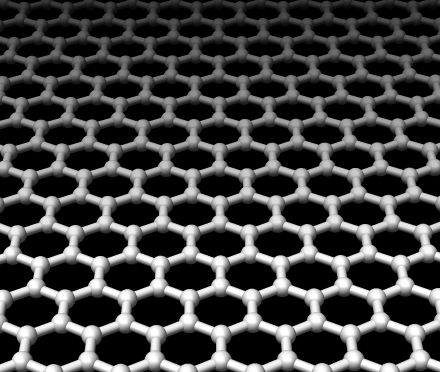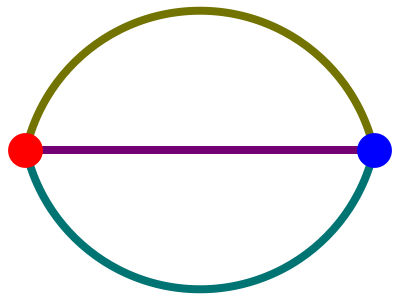## Topological Crystals (Part 1)

A while back, we started talking about crystals:

• John Baez, Diamonds and triamonds, Azimuth, 11 April 2016.

In the comments on that post, a bunch of us worked on some puzzles connected to ‘topological crystallography’—a subject that blends graph theory, topology and mathematical crystallography. You can learn more about that subject here:

• Tosio Sunada, Crystals that nature might miss creating, Notices of the AMS 55 (2008), 208–215.

I got so interested that I wrote this paper about it, with massive help from Greg Egan:

• John Baez, Topological crystals.

I’ll explain the basic ideas in a series of posts here.

First, a few personal words.

I feel a bit guilty putting so much work into this paper when I should be developing network theory to the point where it does our planet some good. I seem to need a certain amount of beautiful pure math to stay sane. But this project did at least teach me a lot about the topology of graphs.

For those not in the know, applying homology theory to graphs might sound fancy and interesting. For people who have studied a reasonable amount of topology, it probably sounds easy and boring. The first homology of a graph of genus$g$ is a free abelian group on$g$ generators: it’s a complete invariant of connected graphs up to homotopy equivalence. Case closed!

But there’s actually more to it, because studying graphs up to homotopy equivalence kills most of the fun. When we’re studying networks in real life we need a more refined outlook on graphs. So some aspects of this project might pay off, someday, in ways that have nothing to do with crystallography. But right now I’ll just talk about it as a fun self-contained set of puzzles.

I’ll start by quickly sketching how to construct topological crystals, and illustrate it with the example of graphene, a 2-dimensional form of carbon:I’ll precisely state our biggest result, which says when this construction gives a crystal where the atoms don’t bump into each other and the bonds between atoms don’t cross each other. Later I may come back and add detail, but for now you can find details in our paper.

### Constructing topological crystals

The ‘maximal abelian cover’ of a graph plays a key role in Sunada’s work on topological crystallography. Just as the universal cover of a connected graph$X$ has the fundamental group$\pi_1(X)$ as its group of deck transformations, the maximal abelian cover, denoted$\overline{X},$ has the abelianization of$\pi_1(X)$ as its group of deck transformations. It thus covers every other connected cover of$X$ whose group of deck transformations is abelian. Since the abelianization of$\pi_1(X)$ is the first homology group$H_1(X,\mathbb{Z}),$ there is a close connection between the maximal abelian cover and homology theory.

In our paper, Greg and I prove that for a large class of graphs, the maximal abelian cover can naturally be embedded in the vector space$H_1(X,\mathbb{R}).$ We call this embedded copy of$\overline{X}$ a ‘topological crystal’. The symmetries of the original graph can be lifted to symmetries of its topological crystal, but the topological crystal also has an$n$-dimensional lattice of translational symmetries. In 2- and 3-dimensional examples, the topological crystal can serve as the blueprint for an actual crystal, with atoms at the vertices and bonds along the edges.

The general construction of topological crystals was developed by Kotani and Sunada, and later by Eon. Sunada uses ‘topological crystal’ for an even more general concept, but we only need a special case.

Here’s how it works. We start with a graph$X.$ This has a space$C_0(X,\mathbb{R})$ of 0-chains, which are formal linear combinations of vertices, and a space$C_1(X,\mathbb{R})$ of 1-chains, which are formal linear combinations of edges. There is a boundary operator$\partial \colon C_1(X,\mathbb{R}) \to C_0(X,\mathbb{R})$

This is the linear operator sending any edge to the difference of its two endpoints. The kernel of this operator is called the space of 1-cycles,$Z_1(X,\mathbb{R}).$ There is an inner product on the space of 1-chains such that edges form an orthonormal basis. This determines an orthogonal projection$\pi \colon C_1(X,\mathbb{R}) \to Z_1(X,\mathbb{R})$

For a graph,$Z_1(X,\mathbb{R})$ is isomorphic to the first homology group$H_1(X,\mathbb{R}).$ So, to obtain the topological crystal of$X,$ we need only embed its maximal abelian cover$\overline{X}$ in$Z_1(X,\mathbb{R}).$ We do this by embedding$\overline{X}$ in$C_1(X,\mathbb{R})$ and then projecting it down via$\pi.$

To accomplish this, we need to fix a basepoint for$X.$ Each path$\gamma$ in$X$ starting at this basepoint determines a 1-chain$c_\gamma.$ These 1-chains correspond to the vertices of$\overline{X}.$ The graph$\overline{X}$ has an edge from$c_\gamma$ to$c_{\gamma'}$ whenever the path$\gamma'$ is obtained by adding an extra edge to$\gamma.$ This edge is a straight line segment from the point$c_\gamma$ to the point$c_{\gamma'}.$

The hard part is checking that the projection$\pi$ maps this copy of$\overline{X}$ into$Z_1(X,\mathbb{R})$ in a one-to-one manner. In Theorems 6 and 7 of our paper we prove that this happens precisely when the graph$X$ has no ‘bridges’: that is, edges whose removal would disconnect$X.$

Kotani and Sunada noted that this condition is necessary. That’s actually pretty easy to see. The challenge was to show that it’s sufficient! For this, our main technical tool is Lemma 5, which for any path$\gamma$ decomposes the 1-chain$c_\gamma$ into manageable pieces.

We call the resulting copy of$\overline{X}$ embedded in$Z_1(X,\mathbb{R})$ a topological crystal.

Let’s see how it works in an example!

Take$X$ to be this graph:Since$X$ has 3 edges, the space of 1-chains is 3-dimensional. Since$X$ has 2 holes, the space of 1-cycles is a 2-dimensional plane in this 3-dimensional space. If we consider paths$\gamma$ in$X$ starting at the red vertex, form the 1-chains$c_\gamma,$ and project them down to this plane, we obtain the following picture:Here the 1-chains$c_\gamma$ are the white and red dots. These are the vertices of$\overline{X},$ while the line segments between them are the edges of$\overline{X}.$ Projecting these vertices and edges onto the plane of 1-cycles, we obtain the topological crystal for$X.$ The blue dots come from projecting the white dots onto the plane of 1-cycles, while the red dots already lie on this plane. The resulting topological crystal provides the pattern for graphene:That’s all there is to the basic idea! But there’s a lot more to say about it, and a lot of fun examples to look at: diamonds, triamonds, hyperquartz and more.

Part 1 – the basic idea.

Part 2 – the maximal abelian cover of a graph.

Part 3 – constructing topological crystals.

Part 4 – examples of topological crystals.

### 10 Responses to Topological Crystals (Part 1)

1.Tom Holroyd says:

You might enjoy this.

http://kurage.nimh.nih.gov/tomh/HCA/

Some of your graphs have negative curvature. In hyperbolic space, there’s enough room to do some interesting things. NP complete problems can be solved in polynomial time, at the expense of exponentially expanding space.

https://www.amazon.com/Cellular-Automata-Hyperbolic-Spaces-Theory/dp/1933153040

And a random interesting paper here:

Click to access 1309.1271.pdf

I don’t understand this stuff much, but tilings of hyperbolic space allow one to see some of the connections between graphs and computation.

2.davetweed says:

I don’t think you need to feel guilty: lots of maths has unexpected applications once people get hold of it. It wouldn’t surprise me if this were eventually to happen to topological crystals.

•John Baez says:

Thanks. It’s a piece of math so simple and natural it just had to be done. But I’m trying to push ahead on network theory to the point where the results are beautiful enough to satisfy my cravings while also making contact with the complexity of living systems. This is tough, because living systems seem “messy”. I feel they are simply operating at a level of abstraction we’re not yet able to handle mathematically.

3.Noam Zeilberger says:

Just a small question: what do you mean by genus of a graph? I’m a bit confused because in this sense of graph genus, the example graph$X$ has genus 0 (rather than 2).

•John Baez says:

I just meant the number of holes. In other words, the dimension of$H^1(X,\mathbb{R})$ is two. I’ll fix that.

4.Noam Zeilberger says:

Should that be $Z_1(X,\mathbb{R}) = Z_1(X,\mathbb{Z}) \otimes R$ in the equation on p.9 of the paper?

•John Baez says:

Thanks! Greg caught that typo a while ago, but apparently I didn’t put the fixed version online. It’s fixed now, and there are some other improvements too.

By the way, to get LaTeX to work in comments here, follow the directions that appear in boldface above the box where you type in your comment.

5. We’re building crystals, like diamonds, purely from topology. Last time I said how: you take a graph$X$ and embed its maximal abelian cover into the vector space$H_1(X,\mathbb{R}).$ Now let me say a bit more about the maximal abelian cover. It’s not nearly as famous as the universal cover, but it’s very nice.

6.Ralph Kaufmann says:

You also might be interested in the paper

• Ralph M. Kaufmann, Sergei Khlebnikov, and Birgit Wehefritz-Kaufmann, The geometry of the Double Gyroid wire network: Quantum and Classical, Journal of Noncommutative Geometry 6 (2012), 623–664.

and subsequent papers.

•John Baez says:

Interesting—thanks!

This site uses Akismet to reduce spam. Learn how your comment data is processed.